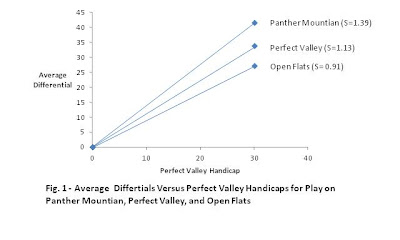## Monday, February 4, 2013

### The Mathematical Underpinnings of the "New" Slope Rating

To see  how the “new” Slope Rating is related to the USGA’s Slope Rating, it is necessary to review the origins of the USGA’s Slope System.  The theory behind the Slope System was first put forward by R.C. Stroud and L.J. Riccio in an article entitled Mathematical underpinning so the slope handicap system.  Stroud starts with Figure 1 below that purports to show how the average unadjusted differential of players from Perfect Valley vary by course.Perfect Valley is depicted as a course of moderate difficulty.  Stroud assigns Perfect Valley a slope of 1.13.    The slope of 1.13 is due to three factors embedded in the handicap system: 1) using only the best 10 of the last 20 differentials, 2) Equitable stroke control, and 3) the .96 bonus for excellence factor. This gives the illusion that an average course would have a Slope Rating of 1.13.  This would not be correct.  Whatever course Stroud selected as his reference course would have a Slope Rating of 1.13.  For example, assume Stroud picked Panther Mountain as his reference course.  The slope of the line relating Average Differential and Panther Mountain Handicap would still be 1.13.  The slope relating the Average Score at Perfect Valley with Panther Mountain Handicap would be 0.92  (i.e., 1.13x1.13/1.39) and the slope at Open Flats would be 0.74.

What should a player’s Standard Course Handicap (i.e., Perfect Valley Handicap or what the USGA now terms a player’s "Index") be at other courses? For generality, let us name the course in question “Any Course.”  The handicap formula tells us:

1)    Standard Handicap(Any Course) = ATBD(Any Course) x .96
Where,
ATBD(Any Course) = Average of Ten Best Differentials at Any Course
The player’s Course Handicap at Any Course can be written as,

2)    Course Handicap(Any Course) = ATBD(Any Course)/1.13

The ATBD(Any Course) is related to his Standard Handicap by eq. 3,

3)    ATBD(Any Course) x .96 = Slope Rating(Any Course) x Standard Handicap
Where,
Slope Rating(Any Course) = Slope of the line relating average differentials at Any  Course with Standard Course handicaps
Substituting eq. 3 into eq. 2, the Handicap(Any Course) becomes

4)    Handicap(Any Course) = Slope Rating(Any Course) x Standard Handicap/1.13
In summary, a player’s handicap at Any Course is given by the product of his Standard Course Handicap and Slope Rating(Any Course) divided by 1.13.

The Slope Rating, however, has little intuitive meaning.  A more informative measure of a course is what is termed the “new” Slope Rating. The new Slope Rating is simply the percentage of your Standard Handicap that is allowed at a course.  In essence,

5)    Handicap(Any Course) = New Slope Rating(Any Course) x Standard Handicap
Substituting from eq. 4 into eq. 5, the New Slope Rating can be defined as:

6)    New Slope Rating(Any Course) = Slope Rating(Any Course)/1.13
If the New Slope Rating of a course is 120, for example, a player gets to play at 120 percent of his Standard Handicap.

 Stroud, R.C., Riccio, L.J., “Mathematical underpinnings of the slope handicap system,” Science and Golf, E & F Spon, London, 1990, p. 136.
 Stroud actually plots average score versus handicap in his paper.  But he also claims (op.cit., p. 140):
So the better half of average differentials (ATBD) divided by average score (for any handicap level) is 1.04/1.13 = 0.92.
This is not correct.  A ten-handicap, for example, would have an ATBD of 10.4 (ATBD= Handicap/.96).  His average score would be the Course Rating plus 11.3 (Handicap x 1.13).   If the Course Rating was 72.0, the ratio of ATBD to average score would be 10.4/83.3 = 0.12.  What Stroud probably meant was that the ratio of ATBD to Average Differential (Average Score – Course Rating) is 0.92.  Therefore, his figure has been changed to reflect this correction. The figure also assumes the average differential of a scratch player is zero.  This assumption is not correct, but is built into the Slope Handicap System.
 The myth that 113 is the Slope Rating of an average course was probably started by Dean Knuth, former Director of Handicapping for the USGA, when he wrote:
“The slope of the scores line of an average course has been observed to be 1.13 and USGA Slope Rating is referenced as 113 to deal with whole numbers.” (See Knuth D., “A two parameter golf course rating system,” Science and Golf, E & F Spon, London, 1990, p. 143.)
Knuth’s explanation is either wrong or possibly just not stated with precision.  The Slope Rating is the slope of the regression line of total score versus USGA handicap for players from the reference course (named Perfect Valley in USGA research papers).  The slope of the regression line of score versus handicap for players at their home course will be 1.13 for all courses according to the USGA.
 The handicap of a player at the Standard Course is measured in tenths and is identical to what is now termed a player’s “index.” See “Simplifying the Slope Handicap System,” www.ongolfhandicaps, 2/1/2013.
 The new Slope Rating has the same validity problems as the old Slope Rating.  Both the USGA’s old Slope Rating and the New Slope Rating are based on the same two assumptions: 1) There is linear relationship between average differentials at Any Course and the handicaps at the Standard Course, and 2) Average differentials are 1.13 times a player’s handicaps.  The validity of these assumptions has not been tested in the peer reviewed literature.